# Cancer Classification Model Using Machine Learning

Machine Learning and Data Science in the medical domain deliver solutions to many problems, specifically in the diagnosis sector. This applies to categorizing diseases, relating the disease to the cause, identifying the root cause, etc.

Machine learning can verify some "impossible-to-understand" phenomena due to medication and plays an essential role in overall medical diagnosis and treatment. To update the treatment process, previously unknown patterns can now be observed and analyzed.

Cancer classification is one area where ML can deliver a robust predictive model based on given observations to identify the cancer possibility. In this article, we will develop our Support Vector Classifier model to predict the presence of malignant (cancer-causing cells) or benign cells.

## Key takeaways from this article

In this blog, a famous approach has been used to predict breast cancer among women. We will understand an answer to the following questions in detail:

• What methods are used to predict the Malignant tumor (Cancer cells)?
• What steps are involved in the SVM classifier implementation for predicting breast cancer?
• How can we evaluate our model using the confusion matrix and ROC curve?
• What are the different domains in medical science where machine learning can help?
• Which are the major companies that are contributing to this area?
• Possible interview questions on this topic.

So let's first define our problem statement in detail and proceed ahead to build a solution for that.

## Problem Understanding

Breast cancer is the most common malignancy (Malignant tumor) among women, accounting for women's second chief cause of cancer death. Breast Cancer occurs due to abnormal growth of cells in the breast tissue, commonly referred to as a Tumor. A tumor does not mean cancer. Tumors can be benign (not cancerous), pre-malignant (pre-cancerous), or malignant (cancerous). Tests such as MRI, mammogram, ultrasound, and biopsy are commonly used to diagnose breast cancer.

## FNA Test

The FNA test is a quick and straightforward process of removing specific fluid from the portion where swelling or soreness is involved in the body. When tested, this fluid can be used to form a discretely labeled dataset that can be employed to develop an ML model for breast cancer classification. This data uses certain features with ground truth results from the FNA test that can be used to check malignant cells and can lead to breast cancer in a patient.

Machine learning techniques such as Artificial Neural Networks, Gradient Boost Method, SVM, etc., help collaborate with the clinical data and can be used to predict the case with a great deal of accuracy. In this article, we will build an SVM model for predicting cancer cells based on specific observations. So let's begin the implementation steps.

## Cancer Classification Model Implementation Steps

The dataset we will be using for this purpose is the `load_breast_cancer` dataset from the sklearn library. The breast cancer dataset is a classic and straightforward binary classification dataset that comes inbuilt with the Scikit-learn library. It can be imported using `sklearn.datasets.load_breast_cancer`

``````from matplotlib import pyplot as plt
import numpy as np
from sklearn.svm import SVC
import pandas as pd

``````

### Step 2: Understanding the data

The dataset has a dimension 569 x 32 with each instance a label 'M' or 'B,' where M = malignant, B = benign.

``````cancer_features=pd.DataFrame(dat.data,columns=dat.feature_names)
``````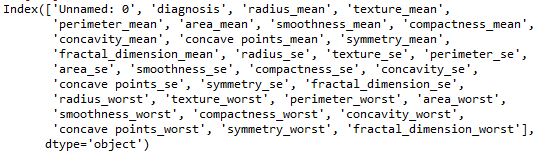The above-shown attributes are the features to be used to predict cancer. Note: The first feature, 'Unnamed: 0', is an index and can be excluded from the final features.

### Step 3: Data Visualization using RedViz

``````from pandas.plotting import radviz
``````

In this dataset, there is a highly non-linear relation between the features, and hence a robust classifier is needed to make any prediction based on it. We have used RadViz ( a non-linear multi-dimensional visualization library) to visualize the dataset of every feature.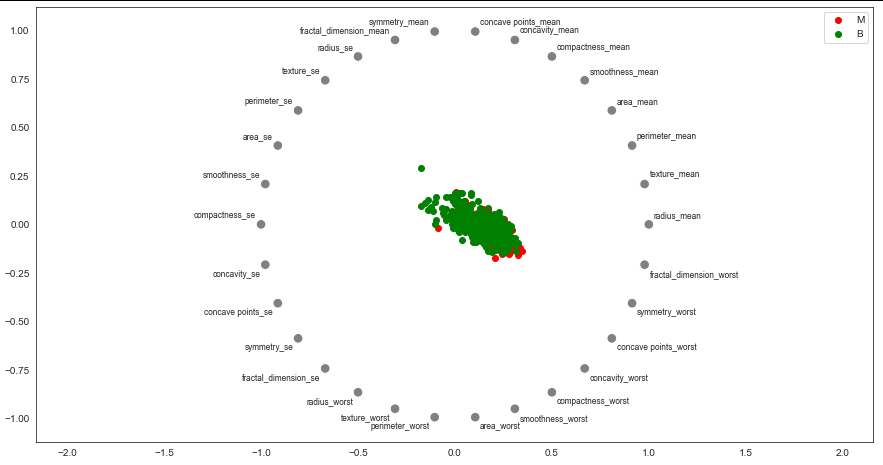RedViz library map the features to a unit circle representation. According to the labels, every instance in the dataset can be seen as 'red' or 'green'. The above visualization clearly shows the high correlation between the dataset instances, making it necessary for a strong classifier to solve this problem.

### Step 4: Data Preprocessing for loadbreastcancer dataset

This step involves several activities such as:

• Assigning numerical values to categorical data (target labels): We can use a label encoder to define the target values in this task. The label encoder can be imported using the command, `from sklearn.preprocessing import LabelEncoder.`Once it is imported, an instance of the label encoder can be created, and the target attribute column (diagnosis) can be fitted.
``````li_classes = [dat.target_names, dat.target_names]
from sklearn.preprocessing import LabelEncoder
le = LabelEncoder()
target_encoded = pd.Series(dat.target)
target = le.fit_transform(target_encoded)
``````
• Standardize every instance of the features: In this step, data standardization can be performed, which will orient the data and a zero mean and unit standard deviation.``````cancer_features=cancer_features.drop(['mean perimeter','mean area','mean radius','mean compactness'],axis=1)
STD=StandardScaler()
cancer_features=STD.fit_transform(cancer_features)
``````

### Step 5: Model Formation

Kernelized support vector machines are robust methods of mapping a highly non-linear dataset to a relatively linear way to classify any dataset instance. Hence we will be using SVM for this task to achieve better performance.

``````from sklearn.model_selection import train_test_split
from sklearn.preprocessing import StandardScaler
from sklearn.model_selection import train_test_split
from sklearn.metrics import accuracy_score
from sklearn.metrics import f1_score
from sklearn.metrics import precision_score
from sklearn.metrics import recall_score
from sklearn.metrics import roc_auc_score
``````
• Obtaining the training and testing data sample: Using the above dataset, we can call the `train_test_split` module from the `sklearn.model_selection` library to divide the dataset into training and testing datasets. The splitting can be done in a 75:25 ratio.
``````x_train,x_test,y_train,y_test=train_test_split(cancer_features,target,test_size=0.25,random_state=0)
``````
• Creating an instance of the model: Import the Support Vector Classifier (SVC) from the SVM module in the sklearn library using `from sklearn.svm import SVC` and creating an instance of the model.
``````model=SVC(C=1.2,kernel='rbf')
model.fit(x_train,y_train)
y_pred=model.predict(x_test)
``````

### Step 6: Overall Pipeline creation

A pipeline can have all the components grouped and executed sequentially. It can be imported from the scikit-learn library as `from sklearn.pipeline import make_pipeline`. This pipeline will take the components incorporated in sequential order and process the input accordingly. The figure below shows that the training dataset is fed to the pipeline. Once the dataset is standardized, it will be provided to the Support Vector Classifier (SVC) to solve a classification problem.

We can use the training set that was prepared earlier and then use `.fit` to fit the training data on the classifier pipeline.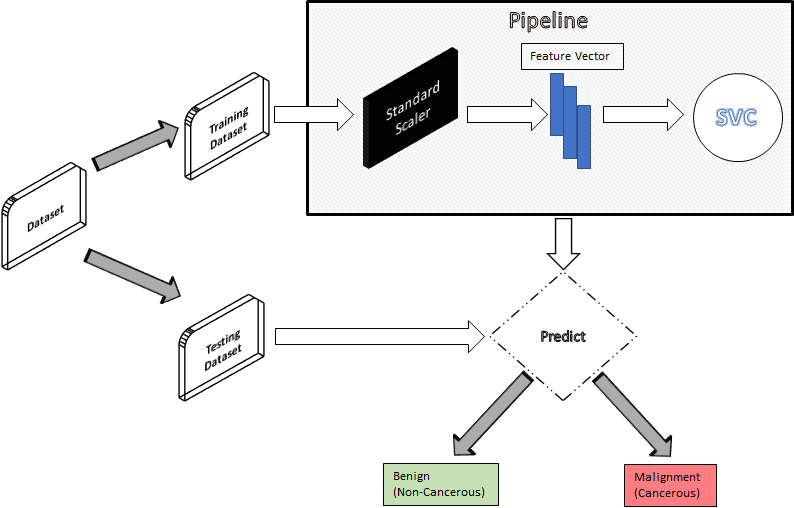The above figure shows the model pipeline to demonstrate the flow. The model used is SVC, which has a lot of tunable parameters, like

• Regularizer, C: By default, it is 1. This parameter is given a positive float quantity, which will inversely relate the regularization's strength to the quantity.
• Kernel: The kernel transforms the data into a different form. The purpose of the kernel is to transform such that the classifier can easily classify it. The most preferred kernel is the 'rbf' because it can account for non-linearity.

The above parameters are of high importance. Other parameters such as the 'gamma' value can be set to auto for the model to take care of itself. Please have a look at the SVM blog for more details.

### Step 7: Performance Evaluation of the SVM model

We have solved a classification problem, so the model can be evaluated on several classification evaluation metrics.

``````print("accuracy: ", accuracy_score(y_test, y_pred))
print("precision: ", precision_score(y_test, y_pred))
print("recall: ", recall_score(y_test, y_pred))
print("f1: ", f1_score(y_test, y_pred))
print("area under curve (auc): ", roc_auc_score(y_test, y_pred))
``````

#### Accuracy Score

This score is simply the percentage of correct prediction in the test set. For the above-given configuration, the accuracy is close to 95.8%.

#### Confusion Matrix

The confusion matrix can be imported from the metrics module of the sklearn library. The test set can compare the predicted output and the ground truth.#### ROC

ROC is the plot between False Positive and True Positive in the plot. It can be imported using. `from sklearn.metrics import roc_curve.`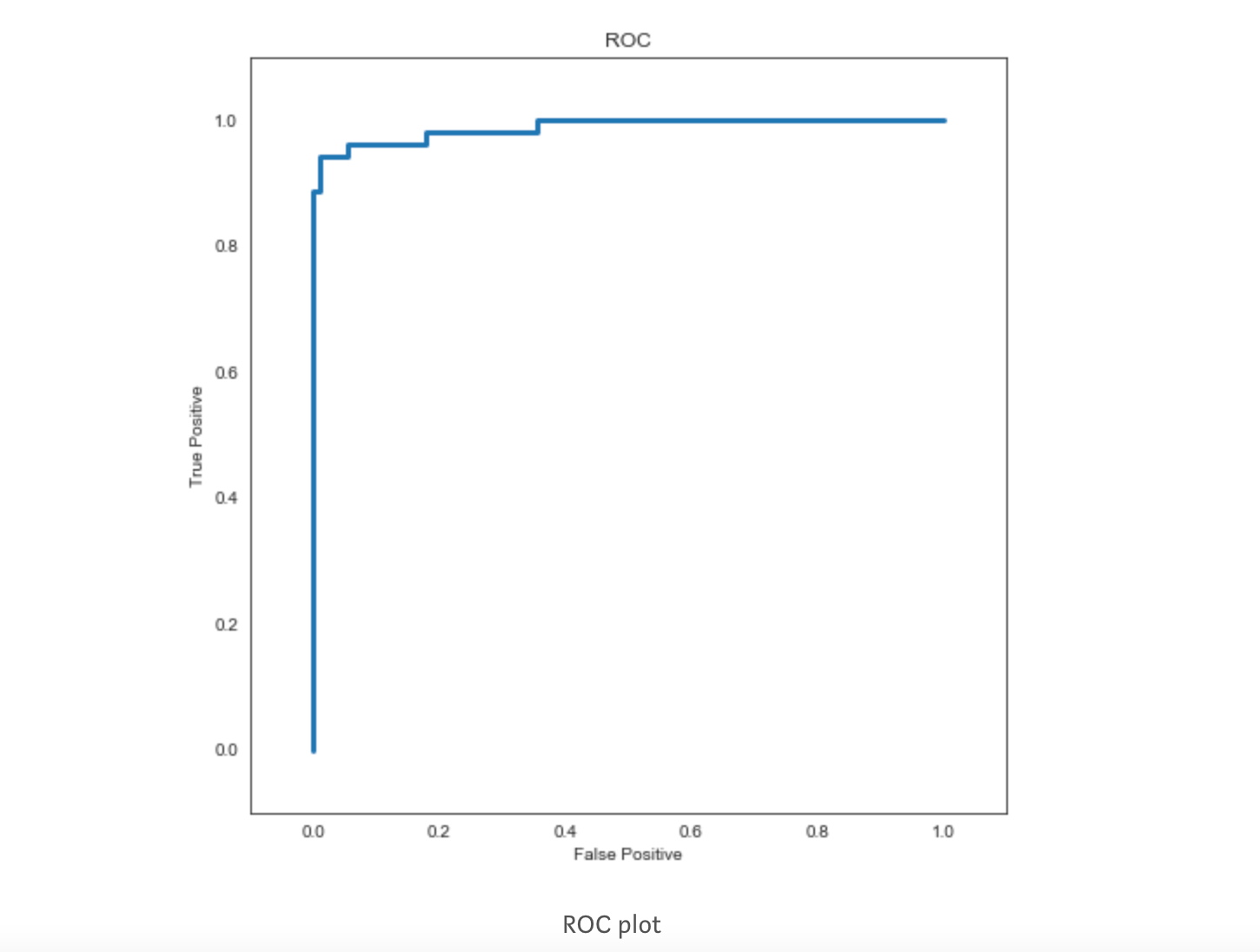We successfully built our model now, and it is performing decently. Now let's see what some other medical science fields where ML can become a boon for us are.

## What are the different domains in medical science where machine learning can help?

ML applications in medical science can range from disease diagnosis to advanced image processing techniques that aid the previously only made by pathologists and microbiologists. Five major areas in the medical domain in which ML is contributing significantly are:

• Disease identification
• Drug discovery
• Smart health records
• Clinical decision making
• Medical imaging Oncology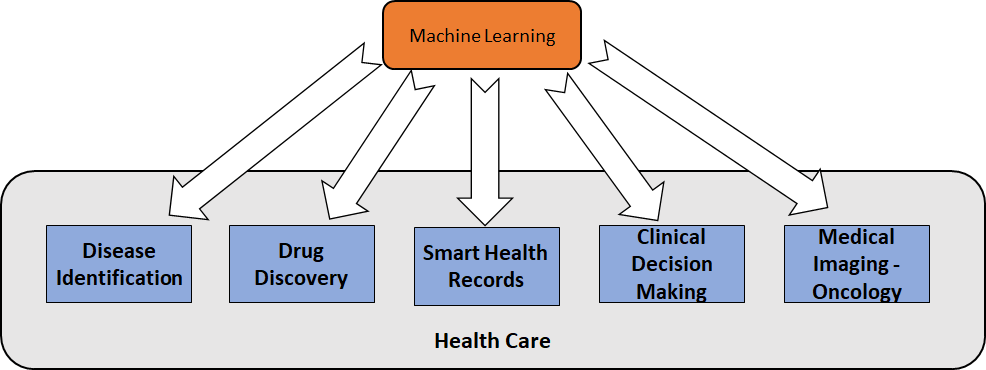Many major companies like Google, IBM, etc., explore machine learning potential in this domain.

## Case studies of Companies Use-case

Early diagnosis of cancer has become a crucial step to saving a life. With this in mind, many top MNCs have invested a significant amount of time and money. Let's have a look at some of these MNCs' work.

Google has taken initiatives to predict cancer's different forms using ML approaches. They have primarily focused on lung cancer, the predominant cause of death, even more than breast cancer. Their algorithm has outperformed radiologists in identifying cancerous cases from CT scan diagnosis images.

### IBM Watson & Mayo Clinic

The two have collaborated. IBM provides its cognitive computing research capabilities, all-inclusive of artificial intelligence, computer vision, and natural language processing, to enable cancerous tissue diagnosis to complement and enhance human expertise in the clinical domain. Mayo Clinic provides the facility for clinical trial data.

The technology helps extract the information more quickly (and painlessly) than any doctor could, identifying patients who best match Mayo Clinic's clinical trial criteria. This involves genomic analysis, matching patients to appropriate clinical trials, and generating evidence to support formal standard-of-care treatment commendations. Watson Health is an example of such cognitive services offered by IBM.

## Possible Interview Questions

If we are going to mention this project in our resumes, then these are some of the possible questions that can be asked in machine learning interviews:

• What are classification problems?
• Why SVM? What other algorithms can be tried in place of SVM.
• What features were used in the final feature set?
• Can we convert this to a multiclass classification problem?
• What more pre-processing of final data can be done?
• What are type-1 and type-2 errors?

## Conclusion

Machine Learning is acting as a lifesaver. It helps doctors identify the disease quickly and provides possible treatments. Breast cancer is a common disease among women that takes more than 4Million lives every year. This number constitutes 14% of the overall death caused by cancer. Machine learning algorithms like SVM, ANNs are perfectly capable of detecting the possibility of breast cancer in patients, which could have taken a long time for doctors to identify. More prominent companies like Google and IBM have invested in cancer identification.

Enjoy Learning, Enjoy Thinking!